Updated date:

# Newton's Law of Universal Gravity

Author:

It is said that one day Newton were siting under an apple tree when suddenly an apple fell on his head. Then Newton asked himself "This apple fell on my head, but why the Moon don't fall on my head?". It was the key question leading Newton to invention of the Universal Law of Gravitation.

This is one version of the famous story about Newton and an apple that inspired him to the great invention. The question is whether the story is true. Many believe that it is only a good anecdote. However, according to the notes and memoirs of his contemporaries, it seems that Newton was really inspired by apples falling on the ground. His acquaintance William Skukeley in his memoirs describes an episode of tea drinking in the garden.

Stukeley attributed the thinking on this occasion "why should that apple always descend perpendicularly to the ground,...why should it not go sideways, or upwards? but constantly to the Earth's center?" to Newton. (Source: Wikipedia, the article "Isaac Newton") Newton's assistant and husband of his niece also described the event in his writing, including Newton's idea that the force causing fall of an apple is the same force that cause Moon's motion around the Earth.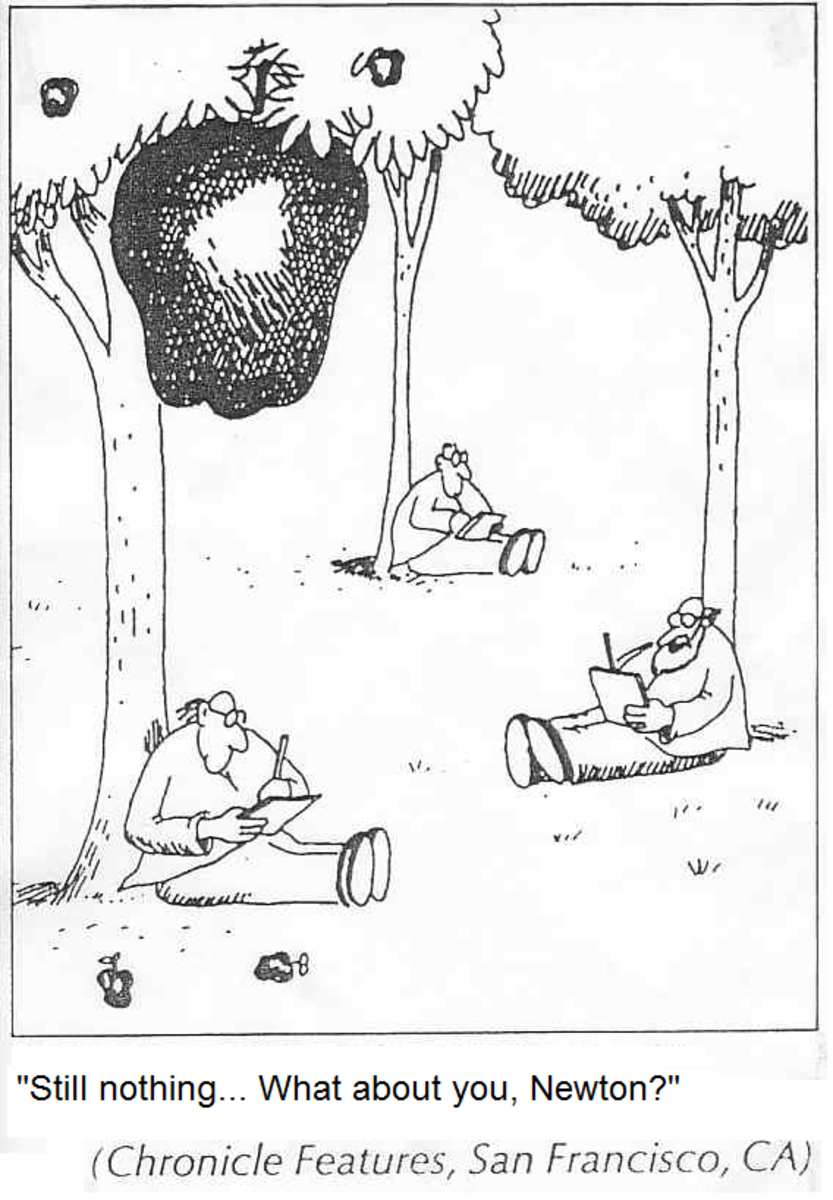Fig. 1. The story of Newton and an apple is inspiration for many.

## How to explain Kepler's laws of planetary motion?

After a German astronomer Johannes Kepler (1571-1630) showed that planets move along an ellipse, a new problem appeared. Why planets move that way? Namely, in that time the circular motion was considered to be a natural movement.

Even Kepler himself tried to answer this question. Kepler assumed that the Sun is a large magnet, and a planet under the influence of the Sun is moving around by magnetic forces.

However, the theory had a huge deficiency. It was known that the Sun is a hot body, and that bodies lose its magnetic properties when they get hot.

Then a French mathematician Rene Descartes (1596-1650) suggest another theory. Descartes proposed a model according to which the entire space is filled with subtle and invisible particles of fluid material. However, Newton later shows that this theory is not in line with Kepler's laws.

Finally, Newton showed that gravitational interaction is the cause of planetary motion. This law, together with the three laws of motion he published in his monumental work "Philosphiae Naturalis Principia Mathematica". He actually solved this problem some twenty years earlier, but it was not published until 1687.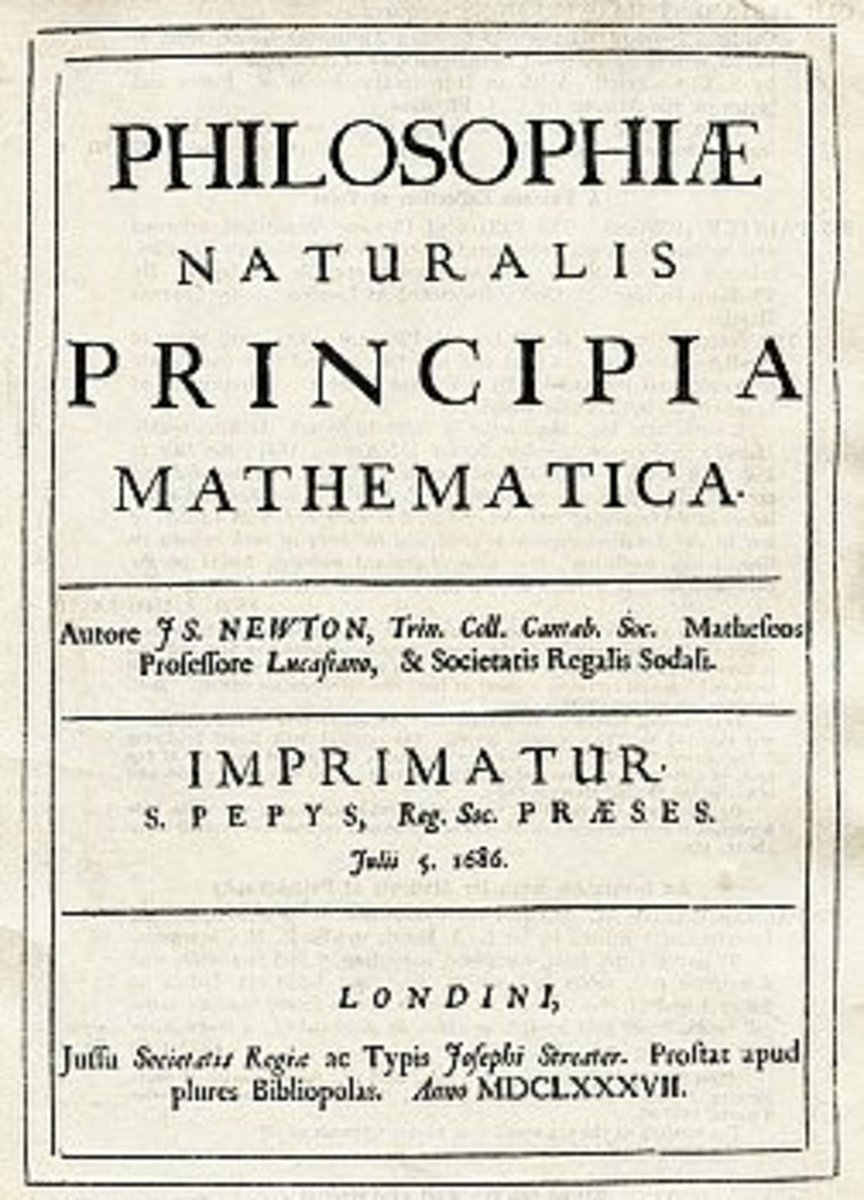Fig. 2. "Principia", one of the most famous scientific books ever. Newton waited for decades to publish his discoveries.

## A brief note on Isaac Newton

• Isaac Newton (1642-1727) enrolled in the study of mathematics at Trinity College, Cambridge.
• Already as a student he made spectacular achievements in mathematics, revealing the binomial theorem and calculus.
• The 1665th in Cambridge, there appeared smallpox and the university was closed. Newton is temporarily returned home to the country.
• During the one-year isolation Newton formulated his most important ideas in mechanics. He formed a clear idea of ​​the first and second law of motion and gravity law.

## Newton's law of universal gravitation

The law of universal gravitation is a physical law derived from empirical observations. Newton was aware, from his first law of motion that some net force had to be acting on the Moon. Otherwise, the Moon would move straightforward instead along an elliptical orbit around the Earth.

Newton's law of universal gravitation states that every two particles in the universe attract each other. That force is proportional to the product of their masses and inversely proportional to the square of the distance between them. More precisely, if masses of particles are m1 and m2 and r is the distance between them then equation in Figure 3 holds. In this relation G is the gravitational constant, whose value is G = 6.673 x 10 -11 Nm2/kg2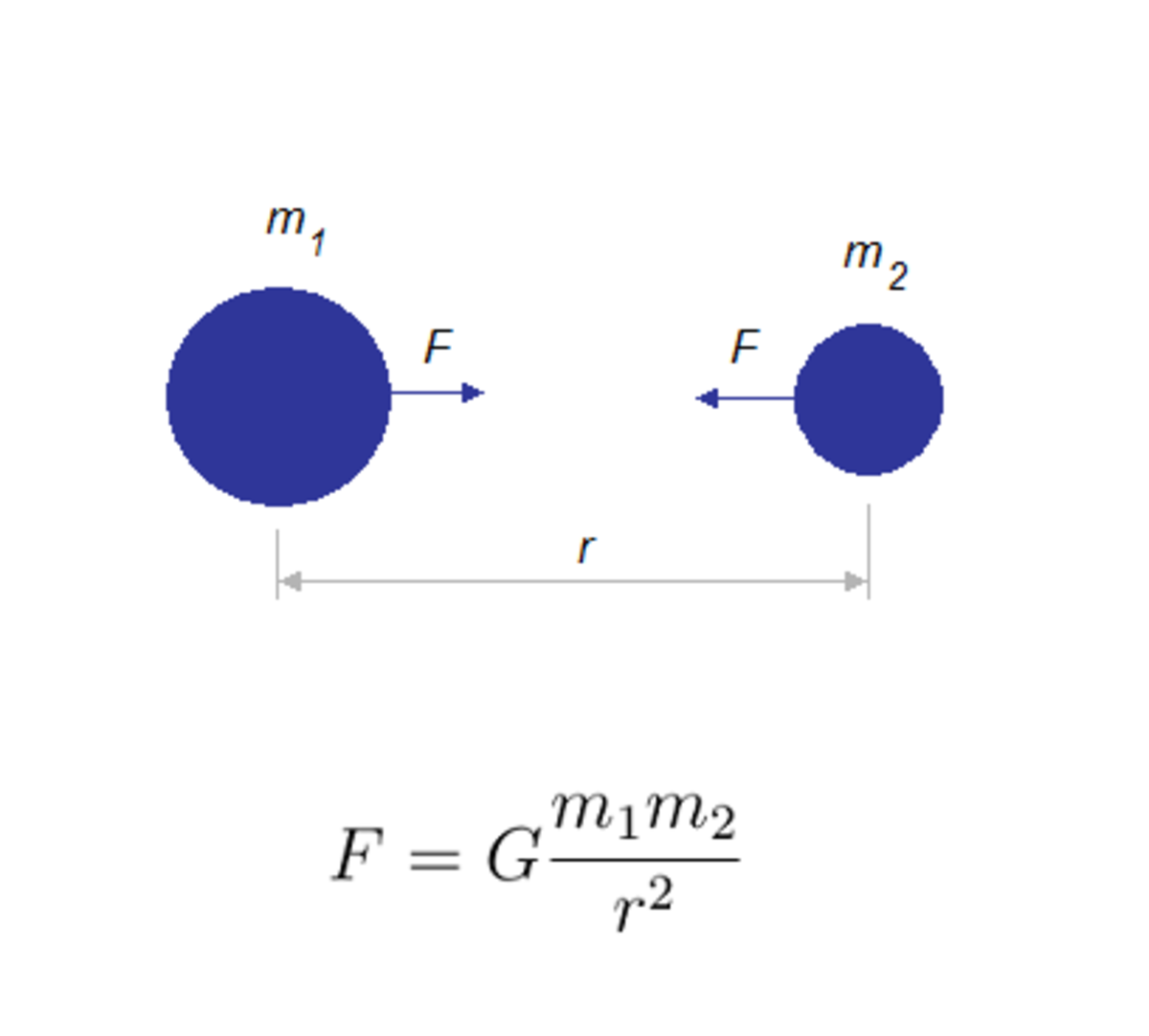Fig. 3. The Newton's law of universal gravitation, a physical law empirically derived.

## Explanation

In order to understand how Newton derived the low, let consider a situation depicted in Figure 4. One can see the Moon orbiting the Earth and an apple falling on the ground (of course, distances are not to scale).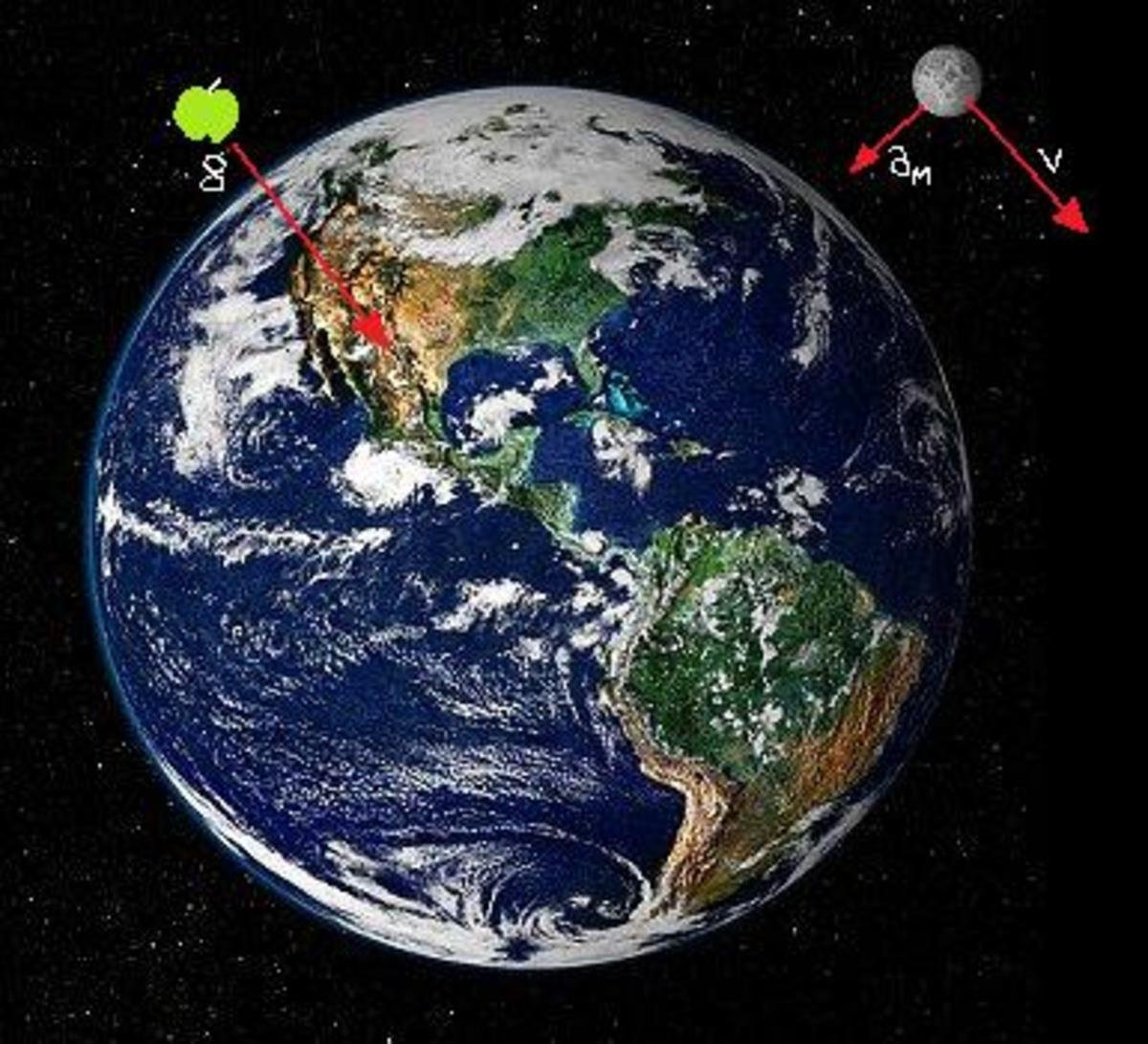Fig. 4. The same force causes the free fall and the circular motion.

For the weight of a body of mass m in the field with gravitational acceleration g it holds F=mg. On the other hand, this is the gravitational interaction between two bodies (a falling body and a planet), thus it holds Newton's law. By equating the two formulas if follows g=GM / r2) where M is the mass of a planet and r its radius (assuming a body is on the surface of planet so planetary radius can be take as a distance).

The centripetal acceleration of the Moon can be calculated knowing the distance from the Earth and orbital period: am=4 π r / T2 . On the other hand the centripetal acceleration can be calculated knowing the above relation for the gravitational acceleration: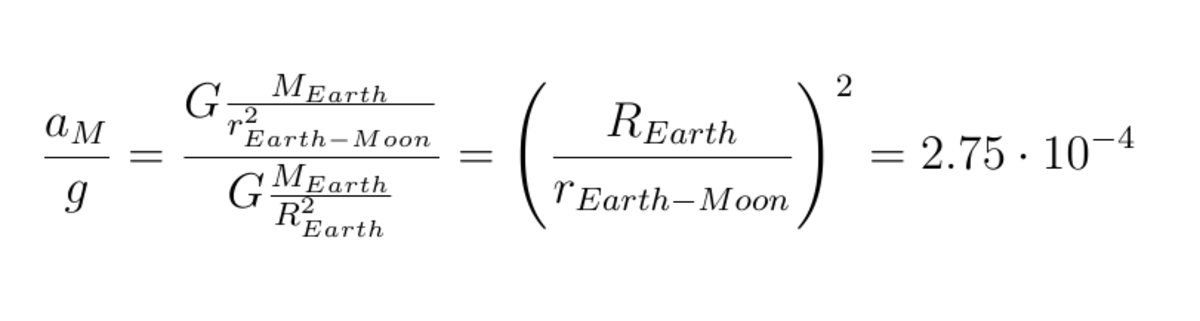It is easy to check that when Newton's law is used, the same result follows as in the fist calculation.

## An Earth satellite

Let return to the first question, from the introduction... indeed, why Moon doesn't fall on the Earth while an apple does. Why in some case the gravity force cause circular motion whereas in the other cases bodies fall on ground? We can answer this question considering energies in the two – object system of a planet and its satellite. Namely, the key difference between the free fall and the circular motion is the velocity of a body.

 Planet, satellite Gravitational acceleration (m/s2) Earth 9.8 Moon 1.63 Mars 3.73 Jupiter 25.93 Pluto 0.61

If the magnitude of velocity is high enough than a satellite will orbit a planet. The minimum speed that the satellite must have at a planet surface in order to move away from is called escape speed. For example, escape speed for the Earth is 11.2 km/s.

Thus, hypothetically, if we throw an apple with at least that speed, it should not dropped but circled around the Earth :-)

## Did you know?

• Newton is known as 'father of second physics', after Aristotle and before Einstein's theory.
• Free fall don't depend on the mass of body which falling. Two bodies of the completely different masses falling from the same height will grounded in the same time. In reality, might not be so because of atmosphere influence.
• Some celestial bodies, within planetoids for instance, have too small mass to be of spherical shape.
• One of the best known Newton's statements is "If I have seen further it is by standing on the shoulders of giants ", from his letter to Robert Hooke (15 February 1676).

## High jump on Mars

As an exercise, let now solve one problem. Mars is the fourth planet from the Sun, known as the red planet (this reddish colour comes from the iron oxide on its surface).

We know that the mass of Earth is 10 times as Mars's mass, while Earth's diameter is twice as Mars's diameter. What would be the "world record" on Mars if Earth's one is 2.45 meter?

Assuming that jump on Mars would be higher than on the Earth as many times the gravitational force is higher on Mars than on Earth, it holds hEarth / hMars = FMars / FEarth. Furthermore, using Newton's law of gravitation as the ratio of heights we have (mMars / RMars2) x (REarth2 / mEarth).

Finally, it holds hEarth / hMars = 4 /10.

Thus, the world record in high jump on Mars would be 6.12 meter.

Video 2. Gravitational interaction is one of the fundamental interactions, that is a force of long distances. The simulation shows a galaxy collision as one of the most spectacular phenomenon caused by gravitational interaction. Two galaxies are of size as Andromeda and Milky Way. Interaction between galaxies means interaction among their stars and other astronomical bodies. There are also photographs of such events.

## Case studies

Comparison of gravitational interaction

Compare the gravitational interaction of the three following cases i.e. systems. Gravitational constant G is 6.67 * 10-11 Nm2kg-2.

a) Earth – Moon. The mass of Earth Me is 6*1024 kg while the mass of Moon Mm is 7.3*1022 kg.The distance r between its centers is 384000 km. What is the interaction F between the Earth and Moon?

Thus, from F = G * Me * Mm / r2 it follows:

F= 6.67 * 10-11 Nm2kg-2 * 6*1024 kg * 7.3*1022 kg / (3.84 * 108 m)2

F = (6.67 * 6 * 7.3 / 3.842 ) * 10-11+24+22-16 N

F = 19.81 * 1019 N = 1.98 * 1019 N

b) Two vessels.What is the gravity between two vessels, 1 km away one of another, if each of them has mass of 107kg?

Again we have the straightforward solution.

F= 6.67 * 10-11 Nm2kg-2 * 1014 kg / 106 m

F= 6.67 * 10-3 N

c) Two neutrons. What is the attractive force between two neutrons on the distance of 10-10 m? The mass of an neutorn is 1.675 * 10-27 kg.

F= 6.67 * 10-11 * 1.6752 *10-54 / 10-20 N

F = 18.71 * 10-11-54+20 N

F = 1.87 * 10-44 N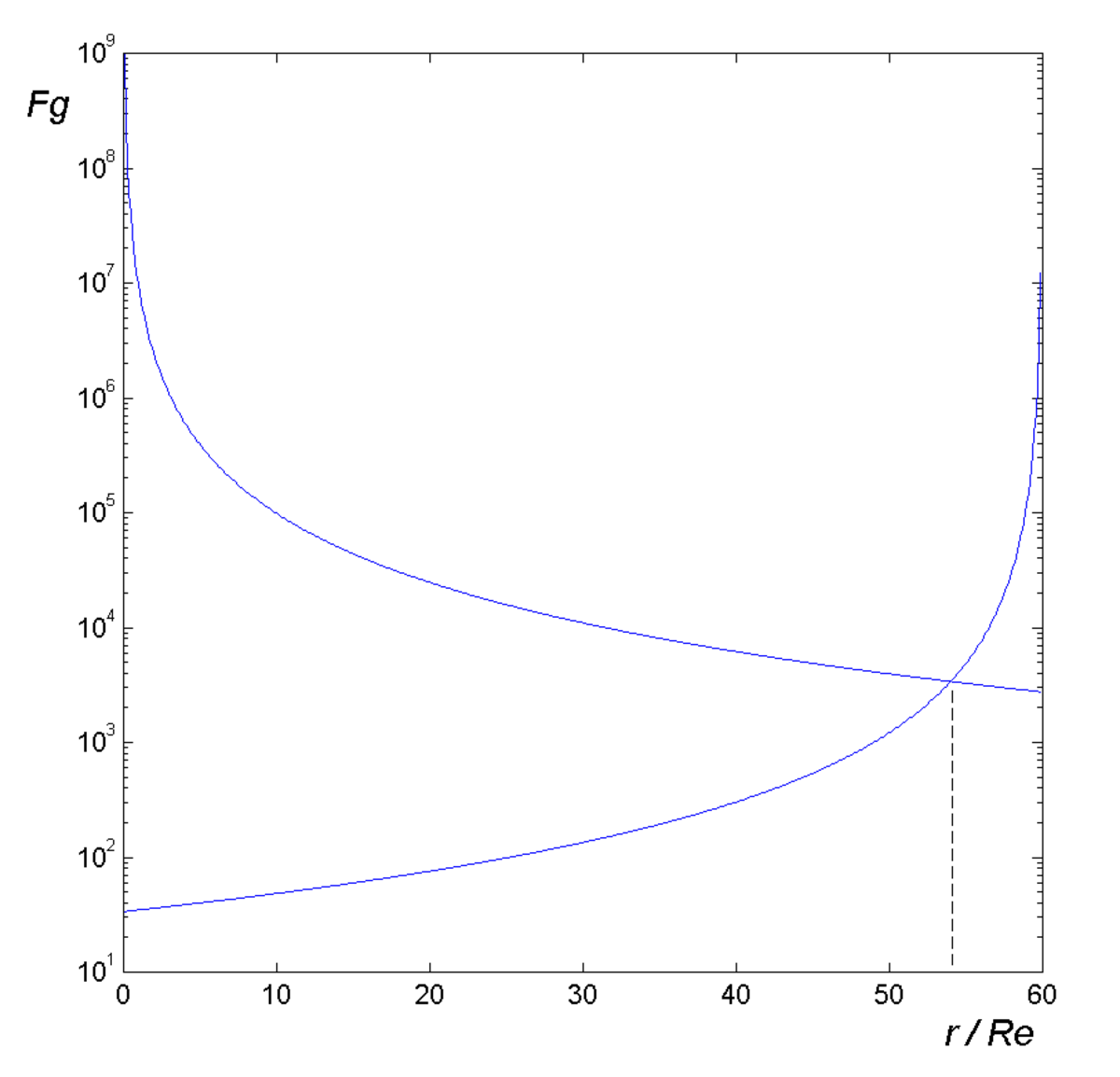Fig. 5. Case study: the point between the Earth and the Moon where the net of the two interactions is zero.

Zero force between the Earth and the Moon

On the line connecting the Earth and the Moon, determine the point where the two attractive forces annul each other. The distance between the Earth and the Moon is 60 Earth's radius, and the mass of Earth is 81 times bigger than the mass of the Moon.

Bodies near the Earth's surface falls on the Earth while bodies on the Moon's surface falls on the its surface. So, we can imagine a point, somewhere between the Earth and the Moon where the net force on a body will be zero. That body wouldn't fall neither to Earth nor Moon. Immediately we can say that the point is closer to the Moon.

Thus, it holds FE = FM which gives G * m* ME / r2= G * m * MM/ r2. When substitute values, we have a quadratic equation, 81 / r2 = 1 / (602 *RE2 – 120 RE * r + r2). A short calculation results with 54RE as the only real solution. The same results we obtain graphically, drawing the force – distance diagram of the two interactions.

## A funny question at the end :-)

For each question, choose the best answer. The answer key is below.

1. A kilogramme of feathers and a kilogramme of iron are falling from the same height. What will be the first to fall ?
• iron, because it has more weight
• in the same time, if there is no atmosphere
• feathers, in any case

### Answer Key

1. in the same time, if there is no atmosphere

© 2012 flysky

## Comments

Sam on December 02, 2013:

such article very science WOW lot of interesting